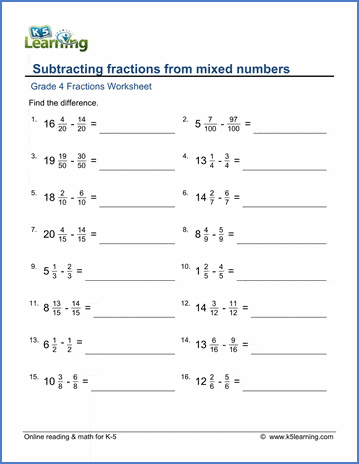# Subtracting fractions from mixed numbers

## Worksheets: Subtract fractions from mixed numbers

Below are six versions of our grade 4 fractions worksheet on subtracting proper fractions from mixed numbers; regrouping will be required in most cases. Answers are given as mixed numbers. These math worksheets are pdf files.## More fractions worksheets

Explore all of our fractions worksheets, from dividing shapes into "equal parts" to multiplying and dividing improper fractions and mixed numbers.

## What is K5?

K5 Learning offers reading and math worksheets, workbooks and an online reading and math program for kids in kindergarten to grade 5.  We help your children build good study habits and excel in school.